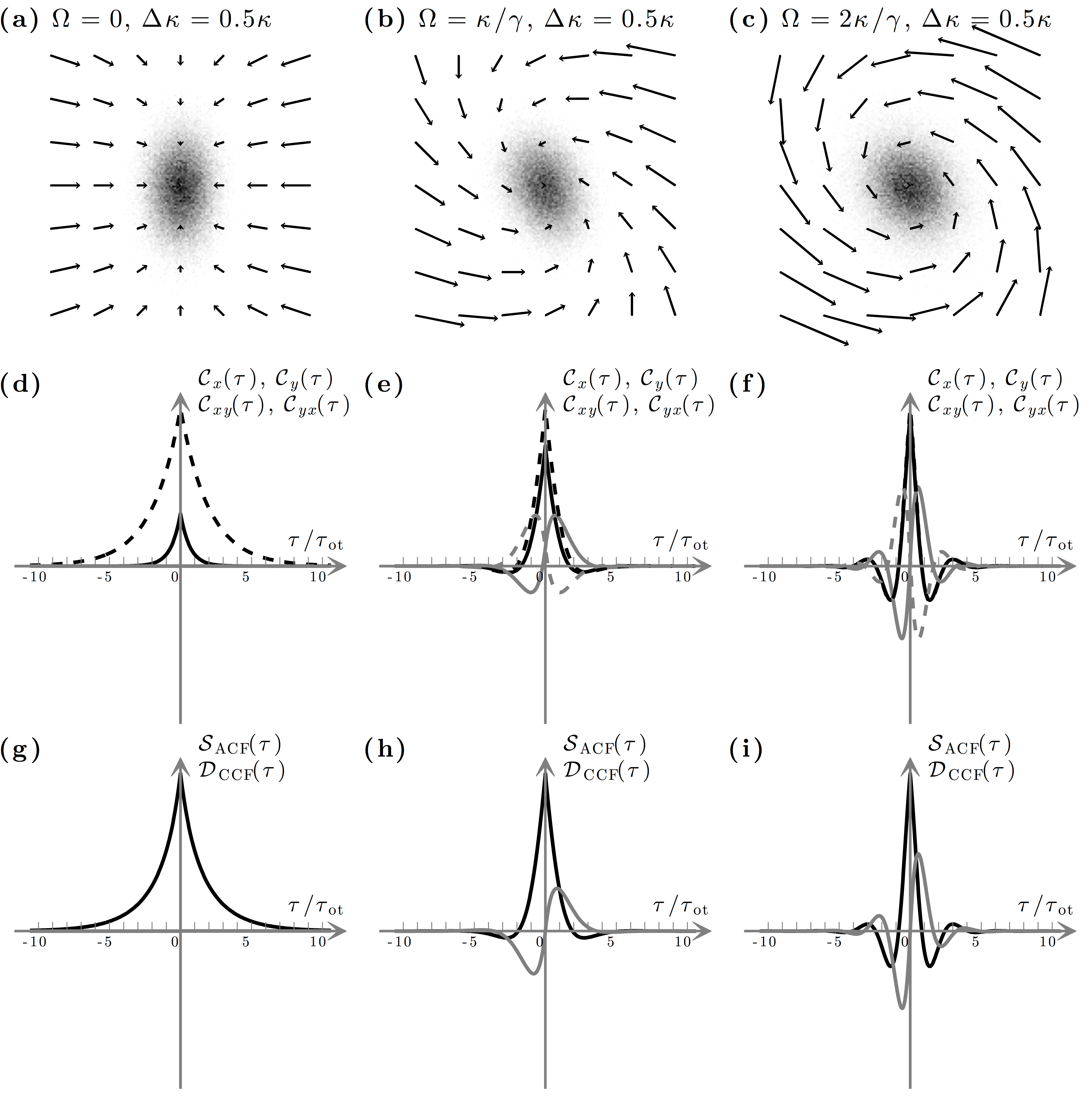# Figure 10.5 — Photonic force microscope in a non-rotationally-symmetric potentialFigure 10.5 — Photonic force microscope in a non-rotationally-symmetric potential. (a)–(c) Photonic torque microscope with a non-rotationally-symmetric harmonic potential, i.e., κx ≠ κy or, equivalently, Δκ ≠ 0, and for various values of Ω. The shaded areas show the probability distribution function of the probe particle position in the corresponding force field (arrows). As the value of Δκ increases, the probability density function becomes more and more elliptical, until for Δκ ≥ κ the probe is confined only along the x-direction and the confinement along the y-direction is lost (not shown). For values of Ω ≥ κ/γ, the rotational component of the force field becomes dominant over the conservative one. The presence of a rotational component masks the asymmetry in the conservative one, since the probability density function assumes a more rotationally symmetric shape. (d)–(f) Corresponding autocorrelation and cross-correlation functions [Eqs. (10.16), (10.17), (10.18) and (10.19)]: Cx (black solid line), Cy (black dashed line), Cxy (grey solid line), and Cyx (grey dashed line). (g)–(i) Corresponding sum (black line) and difference (grey line) [Eqs. (10.23) and (10.24)], which are independent from the choice of the reference system.